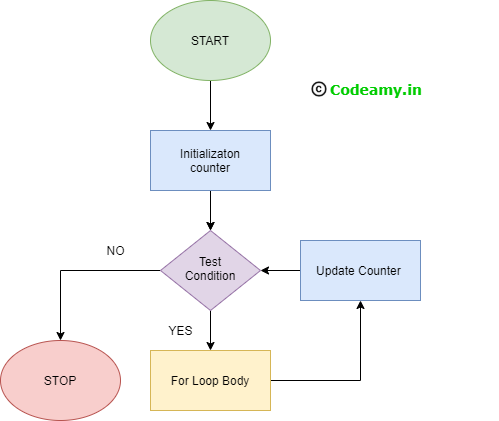# C for Loop - Loops and iteration in C Programming

In this tutorial, we learn about for loop in c programming and understand iteration with examples, syntax, uses

What is the difference between a loop in c programming and an iteration?

Iteration meaning or loop both are the same. This term iteration is used when we use or do the same task again. Let us suppose when someone gives you the task of write one line three times. Then it easy for you to write this but three change to hundred then it is no an easy task for you to do. In normal terms, we use iteration to do the same again.

In programming language provides different inbuilt looping functions. we just use these functions and perform iterative tasks very easily and save time.

## C programming has three types of loops:

1. for loop
2. while loop
3. do...while loop
We learn about while and do...while loop in the next tutorials.

### for Loop Syntax

Here below for loop syntax in c:
``````
for(initialization ; condition ; increment/decreament) {
statement 1;
statement 2;
.
.
.
statement n;
}``````

### How does a for loop work?

for is keyword use for the name for the function and in argument or we talk about three things which are written under ( ) brackets.

Initialization takes an initial or starting value of the loop only once.

The condition is defined here so the compiler understands how many times the block of code repeats. How? Let us suppose the condition is false then the loop is terminated from the loop. If the condition gives result true then the block of statements run and increase/decrease the initial value and check condition for new value and this process repeat again-again until a result come false.

Increment/decrement defined how many times increment/decrement in initial value.

### Flowchartfor Loop

### for loop in c programming example

In this example, we print the sequence of numbers using for loop.
``````
#include <stdio.h>
int main()
{
int i,n;
scanf("%d",&n);
for(i=1 ; i<=n ; i++) {
printf("%d\t",i);
}
return 0;
}
``````

### Explain for Loop Example

1. In "n" takes the endpoint of the sequence of numbers.
2. i=1 is the initialization of i, starting point for the loop.
3. i<=n is the condition for the loop. Let take the user to enter n=10 then the condition is "i<=n" compiler replaced by original values and look like "1<=10". In this condition the result is true. so, print statement print value of i.
4. "i++" increases the value of "i=1 to i=2" and check for condition and if true then again "i" value is an increase.
5. when a condition is false like in case "i=11" then "11<=10" the condition is false and terminate.

### Output

``````
10

1       2       3       4       5       6       7       8       9       10
``````

In the above tutorial, we cover for loop and in the next tutorial learn while or do...while loop in c programming.

Happy Coding 😊

If you have any doubts, Please let me know